# Lecture 6: Basic statistics in a spreadsheet: histograms, frequency, skewness

## Example: Pebbles on a Beach

Let's take an example of the masses of pebbles on a beach. If you pick up a pebble on the beach, it is a specimen. It might not be representative of all the pebbles on the beach (population). But we cannot examine all of the pebbles on the beach, so we select a subset (10 or 100 or 1000, etc.) and that is a sample (dataset) of the population which we hope is representative. Here we will play with a small data set of pebbles. Open this web page in a browser:

http://garnero.asu.edu/glg410/L04_pebbles.html

Below I show a plot of the masses of the individual pebbles in our sample: (a default Excel plot). This chart was made with the Chart Wizard, choosing columns and adding labels, and gives a visual impression of the data, but can we do more? Of course!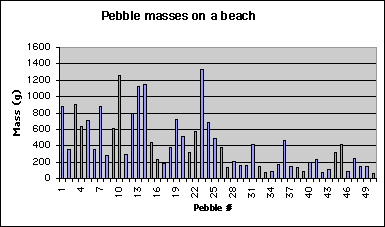## Sorting

The simplest way to look at a dataset is just to sort it. In Excel, look at Data->Sort. You can sort on multiple columns (size, then shape, etc. if they are in your spreadsheet). One really important point is that you should be aware that sorting with only one column will scramble your data. So, you should probably select all of the columns and then in the sort dialogue, choose the column(s) on which you want to sort.

## Introduction

In today's lecture we are continuing our introduction to Microsoft's Excel. We will cover some basic statistics in Excel: histograms and frequency. If your data set is either huge or complicated looking, what is the best approach for analyzing it? Here are some options:

1. Discard all the data that do not fit some preconceived notion or model. For some data sets, this might mean throwing away most of the data! This is very definitely not recommended although it is quite frequently done.
2. Plot the data in a way that allows the general properties of the data to be visualized.
3. Perform statistical (or other scientific) analyses. In other words, examine all the characteristics of the data.

Obviously, we should pursue options 2 and 3. We can apply some statistical tools to Earth and Space science problems. We should be careful however, that a statistical description of our data does not bias our view of the data. So... What is a statistic? --> An (estimated) parameter that characterizes a dataset. Wikipedia.org states:

Statistics is a mathematical science pertaining to the collection, analysis, interpretation, and presentation of data. It is applicable to a wide variety of academic disciplines, from the physical and social sciences to the humanities; it is also used for making informed decisions in all areas of business and government.

Statistical methods can be used to summarize or describe a collection of data; this is called descriptive statistics. In addition, patterns in the data may be modeled in a way that accounts for randomness and uncertainty in the observations, to draw inferences about the process or population being studied; this is called inferential statistics. Both descriptive and inferential statistics can be considered part of applied statistics. There is also a discipline of mathematical statistics, which is concerned with the theoretical basis of the subject.

The word statistics is also the plural of statistic (singular), which refers to the result of applying a statistical algorithm to a set of data. Thus we speak of employment statistics, accident statistics, etc.

When we work with with a large number of observations, it is desirable to be able to describe some of the basic characteristics of the data with single numbers that describe some essential feature of the data, specifically the distribution of numbers. Some important examples are:

• location of the distribution, which characterizes some central point of the "mass" of the distribution (this is commonly referred to as the mean or average)
• dispersion of the mass of the mass about this central value, or a measure of "spread" (you've more likely heard of this as standard deviation)
• skewness of the distribution, which characterizes the asymmetry of the distribution about the central point.

## Measures of location

Here are some basic representations of the mass of the distribution that we'll quickly play with:

Mean = (sum of all values) divided by the number of values
Mode = value which occurs with the greatest frequency
Median = the middle value of a set of numbers arranged in order (half of the numbers have values greater than the median, half are lower.)

For the column of pebble masses, calculate the MEAN, MODE, and MEDIAN. For example, for the mean, do this: in a blank cell, type

=average(J2:J50) {Enter}

where {Enter} denotes hitting the enter key after =average(J2:J50) is typed. Here, J2:J50 is the correct cell range for the pebble masses (this depends on where you pasted the data!). Remember, after you type

=average(

you can simple click and drag in your spreadsheet to encircle the data values, then type the finishing
) {Enter}

Similarly, do the same thing now for mode and median. You should get:
Mean=417.62
Mode=318
Median=881

## Measures of dispersion

Minimum and maximum (MIN and MAX)
Range (= MAX - MIN)
Standard deviation (STDEV) is a measure of dispersion in the sample data: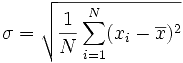Where σ is the standard deviation, N is the number of observations, xi is the ith observation, x with a bar on top is the mean.

± 3 standard deviations always contain more than 90% of the data. Units are the same as the data. Note that this is the square root of the variance (average of the squared distances from the mean).

Relative standard deviation = (STDEV( )/MEAN( ))*100

Thus a relative standard deviation of 10 inches over 1000 feet is different from 10 inches over 20 feet. That is, we are giving relativity to the standard deviation. For the pebble masses, you should get:

Min=62
Max=1336
Range=1274
Standard deviation=335.9393
Relative standard deviation = 80.4414%

## Frequency histograms

How can we improve on the pebbles chart and possibly display the distribution of pebble masses graphically? The simplest method uses the frequency histogram which allows the general properties of the distribution to be visualized.
OLD EXCEL way: If you haven't already done it, install the Analysis Tool Pack [choose Tools>Add Ins.., then check both Analysis Tools Pack boxes], then go to the Tools/Data Anaysis... dialog box, scroll down and choose Histogram.
Office 2007:

1. Click the Microsoft Office Button, and then click Excel Options.
2. Click Add-ins, and then in the Manage box, select Excel Add-ins.
3. Click Go.
4. In the Add-Ins available box, select the Analysis ToolPak check box, and then click OK.

Tip   �If Analysis ToolPak is not listed in the Add-Ins available box, click Browse to locate it.

To construct such a plot, we need to decide on specified ranges of values (bins) within which we want to count the number of pebble masses (Frequency distribution). For example, you might bin masses from 0-100, 100-200, etc. Go ahead and make a column with values 0, 100, then drag the lower right box corner down, which will fill lower cells with that pattern; stop when an appropriate range has been created. Then when we plot the frequency distribution using a bar chart, we have a frequency histogram. Note we might also look at the cumulative frequency distribution in which we add the cumulative percentage of the total specimens that are in each bin. The curvature of the cumulative distribution plot tells us something about the dispersion of the dataset.

Here I will walk you through making this first histogram with a frequency curve, for the pebble data set. You very well may do this dozens of times this semester, so become familiar with these steps. For this example, I'm assuming you already have your pebbles data set correctly entered into a spreadsheet.

1. First, you need to decide on your bin width and range. A bin is the numeric range for which any given bar of the histogram chart adds up. For our pebbles histogram, I think bin ranges of 0-100, 100-200, etc., are appropriate. Make as many bins as you need to completely contain your data. Mine looks like this: (Note: I made labels and shaded labels by right clicking, and choosing Format cells, then click the Patterns tab).

2.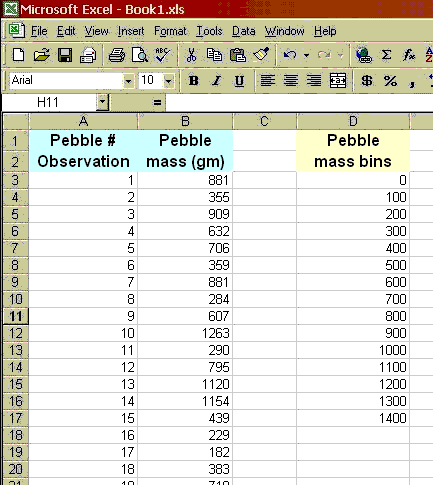3. Now launch the Histogram tool: go to Tools>Data Analysis..., and choose Histogram from the list of tools. You'll see the dialog box wants info about an Input Range ("1" in the diagram below) and a Bin Range (2, below). Click the highlighted square for input range, then click-drag on the spread sheet to encircle your pebble masses.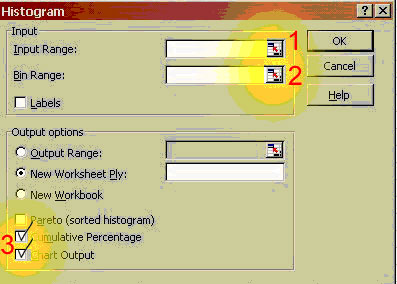You will notice the input window as you do the click-dragging. Then click the button on the right of this window: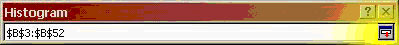Then do the same for Bin Range. Finally, click the Cumulative Percentage and Chart Output options (see "3" in the image on the last page), then OK.
4. You are thrown into a new sheet that should show the following bin, frequency, and cumulative % information: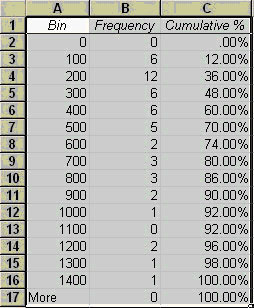The chart is to the right of this, and probably looks something like this: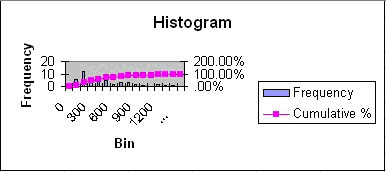Last but not least... we must clean up all of the chart junk, and make this chart more palatable.
After clean up, a plot combining the Frequency histogram with the Cumulative Frequency, using 100 g bins for the histogram looks like: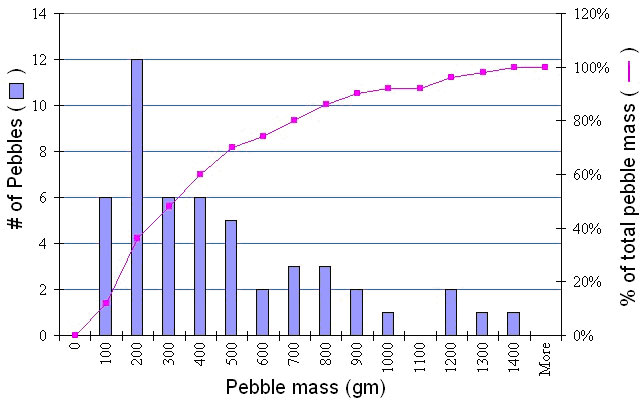## Skewness

Note that the mean is not near the center of the data. These data have significant positive skewness. Positive skewness means that the tail of the distribution is longer to the right. Negative skewness is the opposite. In general, a bigger number means more asymmetry. (be careful that you know what the program is actually computing). Standard skewness is in units of the standard deviation, so if it is <1, then we are close to being symmetric. The skewness is based on the deviations cubed: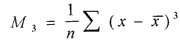Compute the skewness of the pebble distribution:

=SKEW( )

I get a skew of 1.17 which is closer to symmetric than I expected!

Some of the pebbles example comes from short course notes, Statistical inference for Geology and Planetary Geology, L. S. Glaze and S. M. Baloga, 1997; and also: Waltham, D., 1994, Mathematics: a simple tool for geologists, New York: Chapman and Hall, 189 p.

Web page originally by Prof. Ramón Arrowsmith with major modifications and additions from Prof. Ed Garnero and Prof. Steve Semken